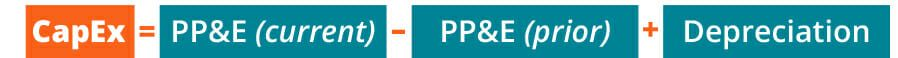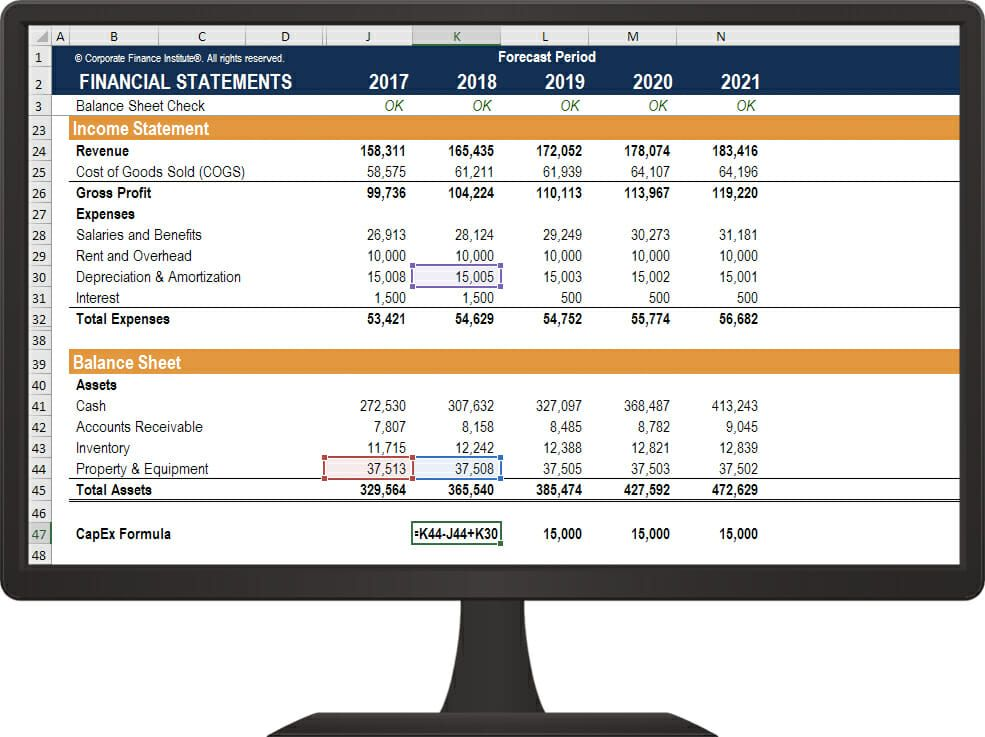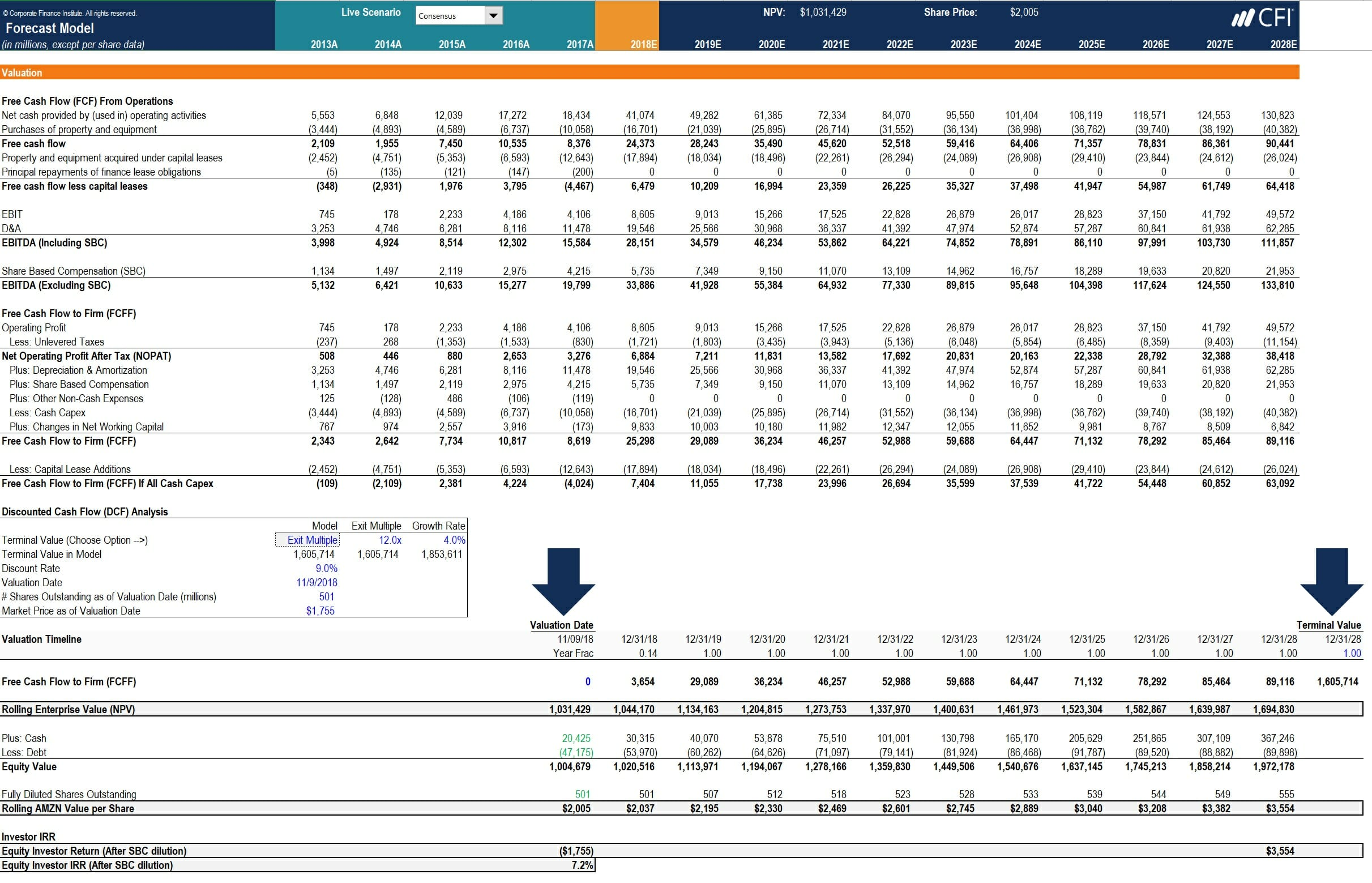# How to Calculate CapEx – Formula

Using the income statement and balance sheet

## What is CapEx?

CapEx (short for capital expenditures) is the money invested by a company in acquiring, maintaining, or improving fixed assets such as property, buildings, factories, equipment, and technology.  CapEx is included in the cash flow statement section of a company’s three financial statements, but it can also be derived from the income statement and balance sheet in most cases.  This guide will provide a formula for how to calculate CapEx.### How to Calculate CapExIf you have access to a company’s cash flow statement, then no calculation is necessary and you can simply see the capital expenditures that were made in the investing cash flow section.

If you don’t have access to the cash flow statement, it’s possible to calculate the net capital expenditure if depreciation is broken out on the income statement (which most, but not all companies do).

To calculate capital expenditures, follow these steps:

1. Locate depreciation and amortization on the income statement
2. Locate the current period property, plant & equipment (PP&E) on the balance sheet
3. Locate the prior period PP&E on the same balance sheet
4. Use the formula below to arrive at CapEx

### CapEx Formula

The CapEx formula from the income statement and balance sheet is:

CapEx = PP&E (current period) – PP&E (prior period) + Depreciation (current period)

This formula is derived from the logic that the current period PP&E on the balance sheet is equal to prior period PP&E plus capital expenditures less depreciation.

Important Note: This formula will produce a “net” capital expenditure number, meaning if there are any dispositions of PP&E in the period, they will lower the value of CapEx that is calculated with the formula.  To adjust for this, you will be required to read the notes to the financial statements. Learn more in CFI’s Financial Analysis Courses.

### Example of the CapEx calculation in Excel

Here is an example of how to calculate capital expenditures, as it applies to financial modeling in Excel:Image: CFI’s Financial Modeling Courses.

In the above example, let’s look at CapEx in 2018 and the following information:

• 2018 Depreciation is \$15,005 on the income statement
• Current period (2018) PP&E is \$37,508 on the balance sheet
• Prior period (2017) PP&E is \$37,513 on the balance sheet

Using the formula provided above, we calculate capital expenditures in 2018 as: \$37,508 – \$37,513 + \$15,005 = \$15,000

### Video Explanation of the CapEx Formula

Below is a short video explanation of how to calculate capital expenditures for a company using only the balance sheet and income statement!

Now try performing the calculation on your own using a real company’s financial statements.

### Applications in Financial Modeling

This CapEx formula can be useful in financial modeling, particularly when working with a company that has complicated financial statements and a lot of detail that goes into their capital asset schedules.

As the below example shows, a net capital expenditures figure can be built to complete the model until more detailed information is provided.